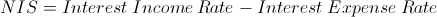The net interest spread formula is used to determine the difference between the rate a bank is earning versus the rate a bank is incurring.

The rate, or yield, that a bank earns and the rate, or yield, that a bank pays is often found in the bank's 10k statement, typically in sections that breakdown the interest income and interest expense portion of the income statement. However, the rate may be monitored on a more short-term basis.

Net interest spread differs from the net interest margin, in that it looks at the rates of income and expenses directly. In contrast, the net interest margin looks more at the rate of return on interest earning income and expenses. Another way of illustrating the differences, is that net interest spread could be considered similar to the "percentage markup" of a product and net interest margin is more similar to "profit margin" in other industries.

## Example of Net Interest Spread

An example of a net interest spread would be to suppose that the average yield for a financial company's assets are 3.5%. If the average yield for the same company's liabilities are 1.5%, the net interest spread would be 2%. Often, within a company's 10-k, a breakdown can be found for the yield for individual assets and for individual liabilities.

## Use of Net Interest Spread

A company would likely keep track of short-term spreads. This information may be coupled with the supply and demand of various interest and non-interest services. Considering that banks offer services that are unrelated to interest, the net interest spread would only be a piece of the larger picture.